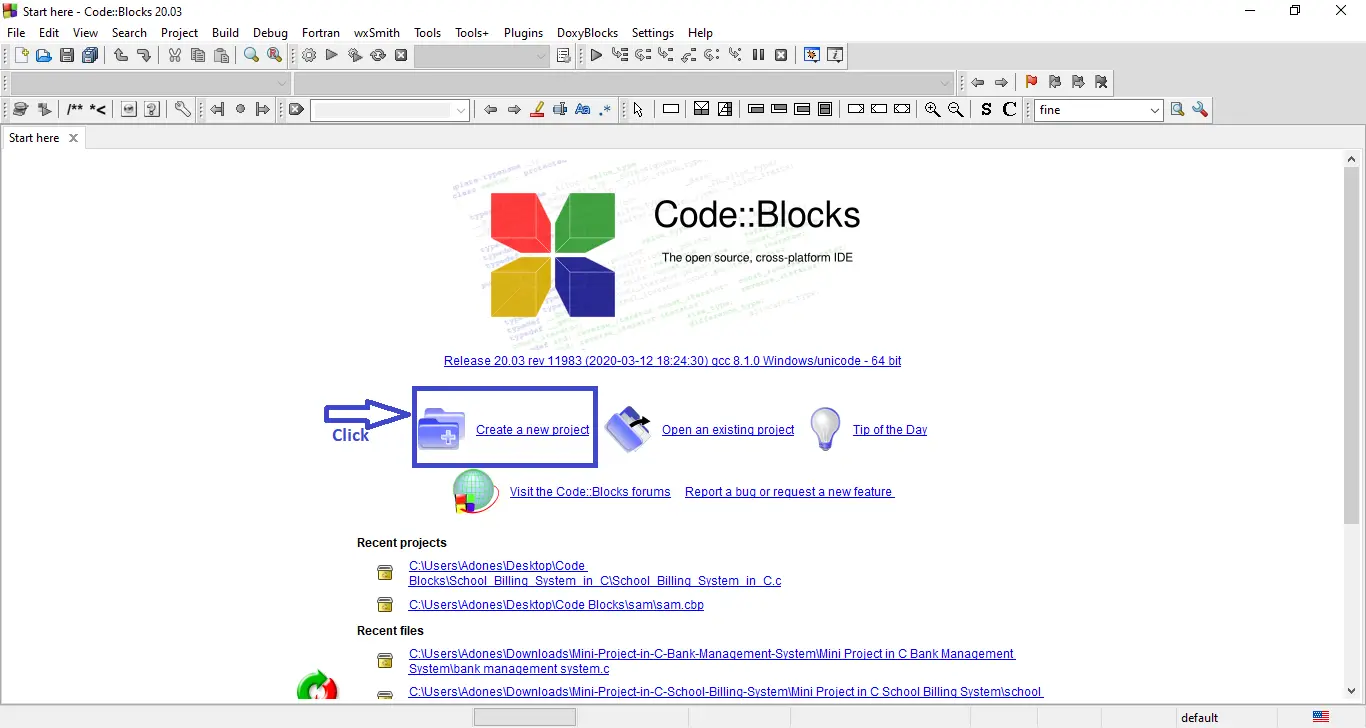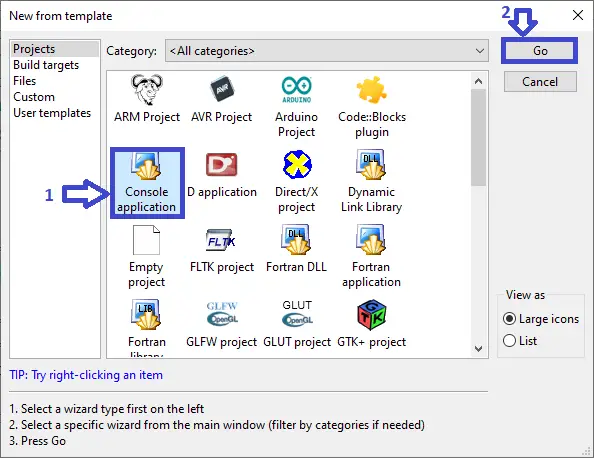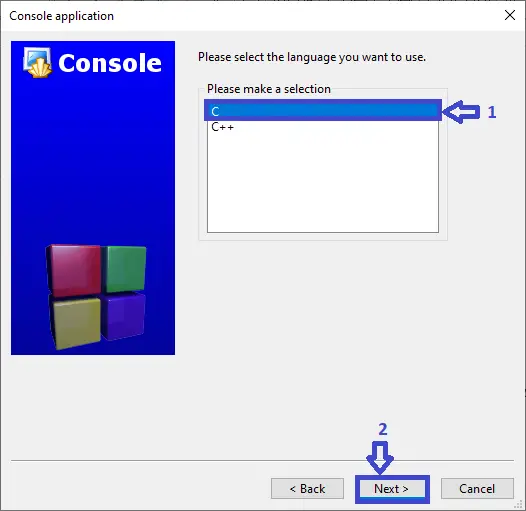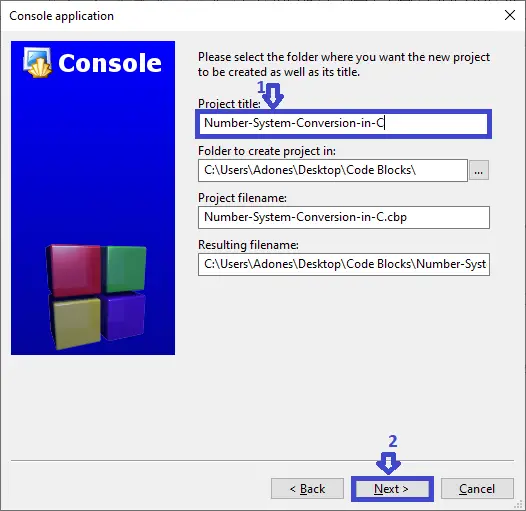# Number System Conversion Project in C with Source Code

This Mini Project for Number System Conversion, the client can effectively change over any number framework such as: binary to decimal, binary to octal, binary to hexadecimal. Decimal to binary, Decimal to octal and Decimal to hexadecimal. Octal to binary, Octal to decimal and Octal to hexadecimal. Hexadecimal to binary, Hexadecimal to decimal and Hexadecimal to octal. The client must select numbers and after that enter the number agreeing to their change. This extend has made the number framework transformation quick and simple for the clients.

Anyway if you want level up your knowledge in programming especially C/C++ Programming Language, try this new article I’ve made for you Best C Projects with Source Code for Beginners Free Download 2022.

Calculator in C Language with Sourc...
Calculator in C Language with Source Code | C Language Projects with Source Code 2021

To start creating a Number System Conversion Project in C , make sure that you have a Code Blocks or any platform of C installed in your computer.

## Steps on how to create a Number System Conversion Project in C

Time needed: 5 minutes.

Number System Conversion Project in C with Source Code

• Step 1: Create a new project.

First open the code blocks IDE and click “create a new project“.• Step 2: Choose console application.

Second click the “console application” and after that click “next“.• Step 3: Choose C language.

Third choose “C language” and click “next“.• Step 4: Name your project.

Fourth name the project you’ve created and click “next” after that click “finish“.• Step 5: The actual code.

## This function is for the main menu

In the code given below, which is for the function to the main menu to choose your own choice of conversion.(Number System Conversion Project in C)

```int main()
{
int operations,numbers=1,checking;
long int b,o,d;
char h;
int x,y,spacebar; // FOR PATTERN
`printf("\t\tWELCOME TO NUMBER SYSTEM CONVERSION\n\n"); while(numbers!=0) { printf("\t\t>>>>>> CHOOSE THE CONVERSION <<<<<<\n\n"); printf("=> BINARY <=\n"); printf("1: Binary to Decimal.\n2: Binary to Octal.\n3: Binary to Hexa-Decimal.\n"); printf("\n=> DECIMAL <=\n"); printf("4: Decimal to Binary.\n5: Decimal to Octal.\n6: Decimal to Hexa-Decimal.\n"); printf("\n=> OCTAL <=\n"); printf("7: Octal to Binary.\n8: Octal to Decimal.\n9: Octal to Hexa-Decimal.\n"); printf("\n=> HEXA-DECIMAL <=\n"); printf("10: Hexa-Decimal to Binary.\n11: Hexa-Decimal to Decimal.\n12: Hexa-Decimal to Octal.\n"); printf("\nENTER YOUR CHOICE: "); scanf("%d",&operations);````

## This function is for the binary

In the code given below, which is for the function for the binary to decimal, binary to octal and binary to hexadecimal.(Number System Conversion Project in C)

```case 1:
printf("\nBINARY TO DECIMAL\n");
D:
printf("\nEnter the Number in Binary form (0s & 1s): ");
scanf("%ld",&b);
// CHECKING INPUT IS IN BINARY FORM
checking=b;
`while(checking!=0) { numbers=checking%10; if(numbers>1) { printf("\n%d IS NOT BINARY NUMBER.\n",b); printf("***TRY AGAIN****\n"); goto D; } else checking=checking/10; } Binary_to_Decimal(b); break; case 2: printf("\n***BINARY TO OCTAL***\n"); E: printf("\nEnter the Number in Binary form (0s & 1s): "); scanf("%ld",&b); // CHECKING INPUT IS IN BINARY FORM checking=b; while(checking!=0) { numbers=checking%10; if(numbers>1) { printf("\n%d IS NOT BINARY NUMBER.\n",b); printf("***TRY AGAIN****\n"); goto E; } else checking=checking/10; } Binary_to_Octal(b); break; case 3: printf("\n***BINARY TO HEXA-DECIMAL***\n"); F: printf("\nEnter the Number in Binary form (0s & 1s): "); scanf("%ld",&b); // CHECKING INPUT IS IN BINARY FORM checking=b; while(checking!=0) { numbers=checking%10; if(numbers>1) { printf("\n%d IS NOT BINARY NUMBER.\n",b); printf("***TRY AGAIN****\n"); goto F; } else checking=checking/10; } Binary_to_Hexadecimal(b); break;````

## This function is for the decimal

In the code given below, which is for the function for Decimal to binary, Decimal to octal and Decimal to hexadecimal.(Number System Conversion Project in C)

```case 4:
printf("\nDECIMAL TO BINARY\n");
printf("\nEnter the Number in Decimal form (0 to 9): ");
scanf("%ld",&d);
Decimal_to_Binary(d); break;
`case 5: printf("\n***DECIMAL TO OCTAL***\n"); printf("\nEnter the Number in Decimal form (0 to 9): "); scanf("%ld",&d); Decimal_to_Octal(d); break; case 6: printf("\n***DECIMAL TO HEXA-DECIMAL***\n"); printf("\nEnter the Number in Decimal form (0 to 9): "); scanf("%ld",&d); Decimal_to_Hexadecimal(d); break;````

## This function is for the octal

In the code given below, which is for the function for Octal to binary, Octal to decimal and Octal to hexadecimal.(Number System Conversion Project in C)

```case 7:
printf("\nOCTAL TO BINARY\n");
A:
printf("\nEnter the Number in Octal form (0 to 7): ");
scanf("%ld",&o);
// CHECKING INPUT IS IN OCTAL FORM
checking=o;
`while(checking!=0) { numbers=checking%10; if(numbers>7) { printf("\n%d IS NOT OCTAL NUMBER.\n",numbers); goto A; } else { checking=checking/10; x++; } } Octal_to_Binary(o); break; case 8: printf("\n***OCTAL TO DECIMAL***\n"); B: printf("\nEnter the Number in Octal form (0 to 7): "); scanf("%ld",&o); // CHECKING INPUT IS IN OCTAL FORM checking=o; while(checking!=0) { numbers=checking%10; if(numbers>7) { printf("\n%d IS NOT OCTAL NUMBER.\n",numbers); goto B; } else { checking=checking/10; x++; } } Octal_to_Decimal(o); break; case 9: printf("\n***OCTAL TO HEXA-DECIMAL***\n"); C: printf("\nEnter the Number in Octal form (0 to 7): "); scanf("%ld",&o); // CHECKING INPUT IS IN OCTAL FORM checking=o; while(checking!=0) { numbers=checking%10; if(numbers>7) { printf("\n%d IS NOT OCTAL NUMBER.\n",numbers); goto C; } else { checking=checking/10; x++; } } Octal_to_Hexadecimal(o); break;````

## This function is for the hexadecimal

In the code given below, which is for the function for Hexadecimal to binary, Hexadecimal to decimal and Hexadecimal to octal.(Number System Conversion Project in C)

```case 10:
printf("\nHEXA-DECIMAL TO BINARY\n");
X:
printf("\nEnter the Number in Hexa-Decimal form: ");
scanf("%s",&h);
//check
for(x=strlen(h)-1;x>=0;x--)
{
if(h[x]>'f' && h[x]<='z' || h[x]>'F'&& h[x]<='Z')
{
printf("\nYou have to Enter Hexa-Decimal Number.\n");
printf("'%c' IS NOT Hexa-Decimal Number.\n",h[x]);
goto X;
}
}
`case 11: printf("\n***HEXA-DECIMAL TO DECIMAL***\n"); Y: printf("\nEnter the Number in Hexa-Decimal form: "); scanf("%s",&h); //check for(x=strlen(h)-1;x>=0;x--) { if(h[x]>'f' && h[x]<='z' || h[x]>'F'&& h[x]<='Z') { printf("\nYou have to Enter Hexa-Decimal Number.\n"); printf("'%c' IS NOT Hexa-Decimal Number.\n",h[x]); goto Y; } } Hexadecimal_to_Decimal(h); break; case 12: printf("\n***HEXA-DECIMAL TO OCTAL***\n"); Z: printf("\nEnter the Number in Hexa-Decimal form: "); scanf("%s",&h); //check for(x=strlen(h)-1;x>=0;x--) { if(h[x]>'f' && h[x]<='z' || h[x]>'F'&& h[x]<='Z') { printf("\nYou have to Enter Hexa-Decimal Number.\n"); printf("'%c' IS NOT Hexa-Decimal Number.\n",h[x]); goto Z; } } Hexadecimal_to_Octal(h); break; default: printf("\n***INVALID NUMBER***\n"); break; } printf("\n\nDO YOU WANT TO CONTINUE = (1/0) :\n"); scanf("%d",&numbers); }````

## Summary

In summary, this 2020 Number System Conversion Project in C project can be useful to students or professional who wants to learn C programming language. This project can also be modified to fit your personal requirements. Hope this project will help you to improve your skills.

That’s how you create Number System Conversion Project in C with Source Code in your projects. You can always expand and try different ways in implementing the Number System Conversion Project in C in your C projects. In this Mini Project for Number System Conversion Project in C is free to download and It is easy to understand and manipulate this project and use for education purpose only.

## Inquiries

### 5 thoughts on “Number System Conversion Project in C with Source Code”

1.ChuyÃªn viÃªn trang Ä‘iá»ƒm sÃ¢n kháº¥u ká»‹ch, Ä‘iá»‡n áº£nh.

2.I really like and appreciate your blog article.Really thank you!

3.Fantastic post. Really Cool.

•4.